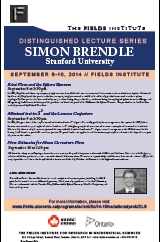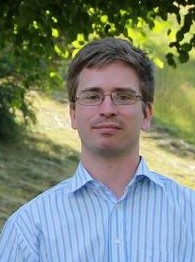# THEMATIC PROGRAMSSeptember 30, 2023THE FIELDS INSTITUTE FOR RESEARCH IN MATHEMATICAL SCIENCES
 Thematic Program on Variational Problems in Physics, Economics and Geometry Distinguished Lecture Series September 8-10, 2014 at 3:30 p.m Fields Institute, Room 230 SIMON BRENDLE Stanford UniversityThematic Program Home Page Video of the talks will be availabe at FieldsLive Distinguished and Coxeter LecturersPhoto: Oberwolfach Photo Collection September 8 at 3:30 pm Ricci Flow and the Sphere Theorem In 1926, Hopf showed that every compact, simply connected manifold with constant curvature 1 is isometric to the standard round sphere. Motivated by this result, Hopf posed the question whether a compact, simply connected manifold with sufficiently pinched curvatured must be a sphere topologically. This question has been studied by many authors during the past decades, a milestone being the topological sphere theorem of Berger and Klingenberg. I will discuss the history of this problem and sketch the proof of the Differentiable Sphere Theorem. The proof relies on the Ricci flow method pioneered by Richard Hamilton." September 9 at 3:30 pm Minimal tori in $S^3$ and the Lawson Conjecture In 1966, Almgren showed that any immersed minimal surface in $S^3$ of genus $0$ is totally geodesic, hence congruent to the equator. In 1970, Blaine Lawson constructed many examples of minimal surfaces in $S^3$ of higher genus; he also constructed numerous examples of immersed minimal tori. Motivated by these results, Lawson conjectured that any embedded minimal surface in $S^3$ of genus $1$ must be congruent to the Clifford torus. In this lecture, I will describe a proof of Lawson's conjecture. The proof involves an application of the maximum principle to a function that depends on a pair of points on the surface." September 10 at 3:30 pm New Estimates for Mean Curvature Flow We describe a sharp noncollapsing estimate for mean curvature flow, which improves earlier work of White and Andrews. This estimate holds for any solution which has positive mean curvature and is free of self-intersections. The estimate is particularly useful for two-dimensional surfaces in $\mathbb{R}^3$; in this case, it provides a substitute for the cylindrical estimates established by Huisken and Sinestrari in the higher-dimensional case. Speakers in the Distinguished and Coxeter Lecture Series have made outstanding contributions to their field of mathematics. The Lecture Sereies consists of three one-hour lectures.